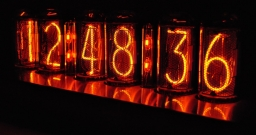# Inverted nine

In the hotel Inverted Nine, each hotel room number is divisible by 6. How many rooms can we count with the three-digit number registered by digits 1,8,7,4,9?

n =  18

### Step-by-step explanation:Did you find an error or inaccuracy? Feel free to write us. Thank you!Tips to related online calculators
Would you like to compute count of combinations?

## Related math problems and questions:

• PermutationsHow many 4-digit numbers can be composed of numbers 1,2,3,4,5,6,7 if: and the digits must not be repeated in the number b, the number should be divisible by five, and the numbers must not be repeated c, digits can be repeated
• Three digits numberFrom the numbers 1, 2, 3, 4, 5 create three-digit numbers that digits not repeat and number is divisible by 2. How many numbers are there?
• Divisible by fiveHow many different three-digit numbers divisible by five can we create from the digits 2, 4, 5? We can repeat the digits in the created number.
• The HotelThe Holiday Hotel has the same number of rooms on each floor. Rooms are numbered with natural numerals sequentially from the first floor, no number is omitted, and each room has a different number. Three tourists arrived at the hotel. The first one was in
• DigitsHow many five-digit numbers can be written from numbers 0.3,4, 5, 7 that is divided by 10, and if digits can be repeated?
• NumbersHow many different 3 digit natural numbers in which no digit is repeated, can be composed from digits 0,1,2?
• CombinationsHow many different combinations of two-digit number divisible by 4 arises from the digits 3, 5 and 7?
• Three-digit numbersWe have digits 0,1,4,7 that cannot be repeated. How many three-digit numbers can we write from them? You can help by listing all the numbers.
• DigitsHow many natural numbers greater than 4000 which are formed from the numbers 0,1,3,7,9 with the figures not repeated, B) How many will the number of natural numbers less than 4000 and the numbers can be repeated?
• Three-digit numbersHow many are all three-digit numbers that are made up of digits 0,2,5,7 and are divisible by 9 if the digits can be repeated?
• Three-digit numbersHow many three-digit numbers are from the numbers 0 2 4 6 8 (with/without repetition)?
• How many 4How many four-digit numbers that are divisible by ten can be created from the numbers 3, 5, 7, 8, 9, 0 such no digits repeats?
• Five-digit numberAnna thinks of a five-digit number that is not divisible by three or four. If he increments each digit by one, it gets a five-digit number that is divisible by three. If he reduces each digit by one, he gets a five-digit number divisible by four. If it sw
• Four-digit numbersHow many four-digit numbers can we make from the numbers 2, 6, 3, 5, 1 and 9 if the numbers in the number cannot be repeated?
• Six-digit primesFind all six-digit prime numbers that contain each one of digits 1,2,4,5,7, and 8 just once. How many are they?
• By sixFrom the digits 1,2,3,4 we create the long integer number 123412341234. .. .. , which will have 962 digits. Is this number divisible by 6?
• Dd 2-digit numbersFind all odd 2-digit natural numbers compiled from digits 1; 3; 4; 6; 8 if the digits are not repeated.